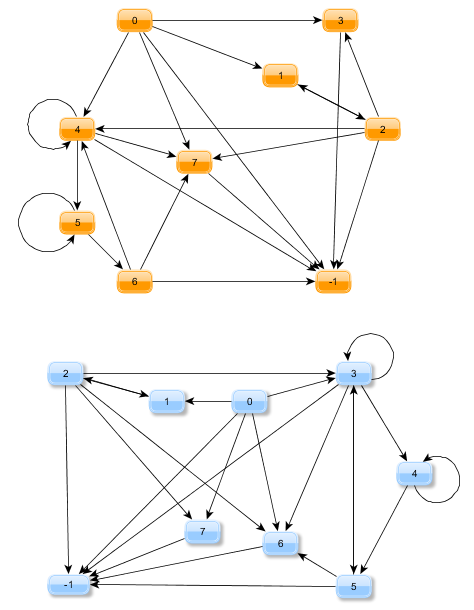## Maptrackers

### From graphs to maps

A Maptracker is a special backtracking algorithm used to check the equivalence of certain maps which can be represented as connected, directed graphs or finite state machines. It shall be described in this article.

The original motivation was to find an algorithm for reconstruction of grammars from finite state-machines with the following property: suppose you have a state-machine M0 and a function P which turns M0 into a grammar rule: G = P(M0). When we translate G back again into a state-machine we get M1 = T(P(M0)). Generally T o P != Id and M0 != M1. But how different are M0 and M1 actually?Watch the two graphs GR1 and GR2 above. When we abstract from their particular drawings and focus on the nodes and edges only we can describe them using the following dictionaries:

```GR1 = {0: set([1, 3, 4, -1, 7]), 1: set(), 2: set([1, 3, 4, -1, 7]), 3: set([-1]), 4: set([6, 4, 5, -1, 7]), 5: set([5, 6]), 6: set([4, -1, 7]), 7: set([-1])}```
```GR2 = {0: set([1, 3, 6, -1, 7]), 1: set(), 2: set([1, 3, 6, -1, 7]), 3: set([6, 3, 4, 5, -1]), 4: set([4, 5]), 5: set([3, 6, -1]), 6: set([-1]), 7: set([-1])}```

A pair i: [j1, j2, … jn] describes the set of edges i -> j1,  i -> j2, …, i -> jn.

### Checking for equivalence

We say that GR1 and GR2 are equivalent if there is a permutation P of  {-1, 0, 1, …, 7} and

`GR2 == dict( (P(key), set(map(P, value))) for (key, value) in GR1.items() )`

Maptracker is merely a cute name for an algorithm which constructs the permutation P from map representations of the kind GR1 and GR2. P itself will be described as a dictionary. Since the value -1 is a fixed point it will be omitted:

```class Maptracker(object): def __init__(self, gr1, gr2): self.gr1 = gr1 self.gr2 = gr2   def accept(self, value, stack): e1, e2 = value # e1 -&gt; e2 V1 = self.gr1[e1] V2 = self.gr2[e2] # # e1 -&gt; e2 =&gt; v1 -&gt; v2 # # check consistency of the choice of the mapping if len(V1)!=len(V2): return False m = dict(p for (p,q) in stack) if e2 in m.values(): return False for v1 in V1: if v1 == e1: if e2 not in V2: return False if v1 in m: if m[v1] not in V2: return False for s in m: if e1 in self.gr1[s]: if e2 not in self.gr2[m[s]]: return False return True   def run(self): stack = [] if len(self.gr1) != len(self.gr2): return {} sig1 = sorted(len(v) for v in self.gr1.values()) sig2 = sorted(len(v) for v in self.gr2.values()) if sig1!=sig2: return {}   L1 = self.gr1.keys() L2 = self.gr2.keys() i = j = 0 while i&lt;len(L1): e1 = L1[i] while j&lt;len(L2): e2 = L2[j] if self.accept((e1,e2),stack): stack.append(((e1,e2),(i,j))) j = 0 break j+=1 else: if stack: _, (i,j) = stack.pop() if j == -1: return {} j+=1 continue else: return {} i+=1 return dict(elem for elem in stack)```

If no permutation could be constructed an empty dictionary {} is returned.

Let’s watch the dict which is computed by the Maptracker for GR1 and GR2:

```&gt;&gt;&gt; M = Maptracker(GR1, GR2).run() &gt;&gt;&gt; M {0: 0, 1: 1, 2: 2, 3: 7, 4: 3, 5: 4, 6: 5, 7: 6}```

We can check the correctness of M manually or by setting

`P = lambda k: (-1 if k == -1 else M[k] )`

and check the equality we have defined above.

1.sateesh says:

I think the dictionary definitions GR1 and GR2 are interchanged. GR1 corresponds to the bottom diagram and GR2 corresponds to the top diagram, was that the intention ?

2.kay says:

@sateesh, you are right. I’ve updated the article accordingly. Thanks!

1. There are no trackbacks for this post yet.